# PERMUTATION & COMBINATION

PERMUTATION & COMBINATION

FUNDAMENTAL PRINCIPLE:

If an event A can occur in 'm' different ways and another event B can occur in 'n' different ways, then the total number of different ways of-

(a)  simultaneous occurrence of both events in a definite order is m n. This can be extended to any number of events (known as multiplication principle).

(b)  happening exactly one of the events is m + n (known as addition principle).

Example :

A college offers 6 courses in the morning and 4 in the evening. The possible number of choices with the student if he wants to study one course in the morning and one in the evening is?

Solution :

The student has 6 choices from the morning courses out of which he can select one course in 6 ways.

For the evening course, he has 4 choices out of which he can select one in 4 ways.
Hence the total number of ways 6 * 4 = 24.

Example :

A college offers 6 courses in the morning and 4 in the evening. The number of ways a student can select exactly one course, either in the morning or in the evening?

Solution :

The student has 6 choices from the morning courses out of which he can select one course in 6 ways.
For the evening course, he has 4 choices out of which he can select one in 4 ways.

Hence the total number of ways 6 + 4 = 10.

PERMUTATION & COMBINATION :

Factorial :

A Useful Notation : n! = n.(n – 1).(n – 2)..............3. 2. 1;

n! = n. (n – 1)! where n is real number.

Points to Remember :

• 0! = 1! = 1
• Factorials of negative integers are not defined.
• (2n)! = 2^n.n! [1. 3. 5. 7........(2n – 1)]

•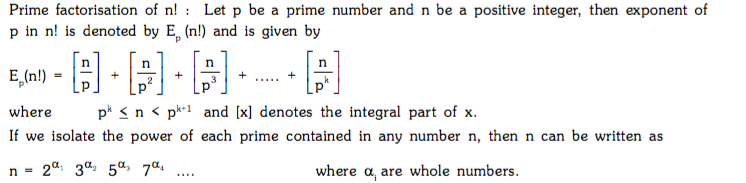Permutation

Each of the arrangements in a definite order which can be made by taking some or all of the things at a time is called a PERMUTATION. In permutation, order of appearance of things is taken into account; when the order is changed, a different permutation is obtained.

Generally, it involves the problems of arrangements (standing in a line, seated in a row), problems on digit, problems on letters from a word etc.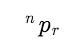denotes the number of permutations of n different things, taken r at a time (n and r real numbers and r<= n)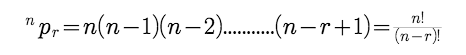Points to Remember: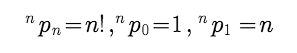Number of arrangements of n distinct things taken all at a time = n!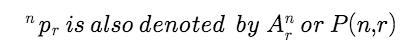Combination

Each of the groups or selections which can be made by taking some or all of the things without considering the order of the things in each group is called a COMBINATION.

Generally, involves the problem of selections, choosing, distributed groups formation, committee formation, geometrical problems etc.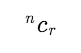nCr denotes the number of combinations of n different things taken r at a time (n => N, r => W, r < n)

Points to remember :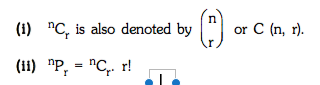Example :

If a denotes the number of permutations of (x + 2) things taken all at a time, b the number of permutations of x things taken 11 at a time and c the number of permutations of (x – 11) things taken all at a time such that a = 182 bc, then the value of x is?

Solution :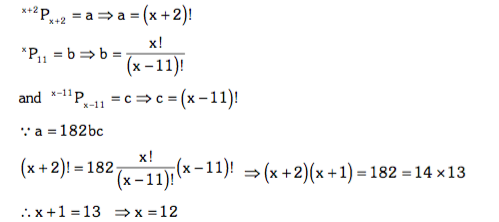Example :

A box contains 5 different red and 6 different white balls. In how many ways can 6 balls be drawn so that there are atleast two balls of each colour ?

Solution :

The selections of 6 balls, consisting of atleast two balls of each colour from 5 red and 6 white balls, can be made in the following ways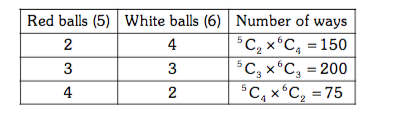Therefore total number of ways = 425

Example :

If all the letters of the word 'RAPID' are arranged in all possible manner as they are in a dictionary,

then find the rank of the word 'RAPID'.

Solution :

First of all, arrange all letters of given word alphabetically : 'ADIPR'

No of word starting with A_ _ _ _  = 4! = 24

No of word starting with D_ _ _ _  = 4! = 24

No of word starting with I_ _ _ _  = 4! = 24

No of word starting with P_ _ _ _  = 4! = 24

No of word starting with RAD_ _   = 2! = 2

No of word starting with RAI_ _   = 2! = 2

No of word starting with RAID _   = 1! = 1

No of word starting with RAPI _   = 1! = 1

Rank of WORD 'RAPID'  = 24+ 24 + 24 +24 + 2 + 2 + 1 + 1 = 102

PERMUTATIONS OF ALIKE OBJECTS

The number of permutations of n things taken all at a time : when p of them are similar of one type, q of them are similar of second type, r of them are similar of third type and the remaining n – (p + q+ r) are all different is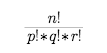Example :

In how many ways the letters of the word "ARRANGE" can be arranged without altering the relative position of vowels & consonants.

Solution :

The consonants in their positions can be arranged in 4!/2! = 12 ways.

The vowels in their positions can be arranged in 3!/2! = 3 ways

so Total number of arrangements = 12*3 = 36

Example :

How many numbers can be formed with the digits 1, 2, 3, 4, 3, 2, 1 so that the odd digits always occupy the odd places?

Solution :

There are 4 odd digits (1, 1, 3, 3) and 4 odd places (first, third, fifth and seventh). At these places

the odd digits can be arranged in 4!/2!*2!  = 6 ways

Then at the remaining 3 places, the remaining three digits (2, 2, 4) can be arranged in 3!/2! = 3 ways

The required number of numbers = 6*3 = 18

Example :

Find the total number of 4 letter words formed using four letters from the word ''PARALLELOPIPED'.

Solution :

Given letters are PPP, LLL, AA, EE, R, O, I, D.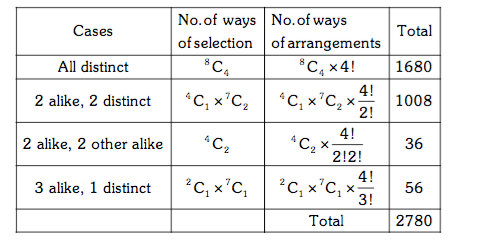Post By : Preeti Rai 01 May, 2019 4606 views Maths Permutation & Combination